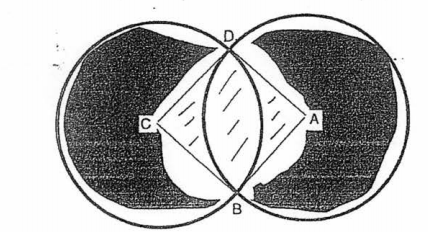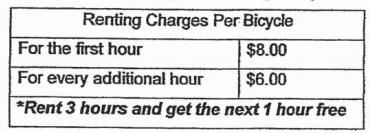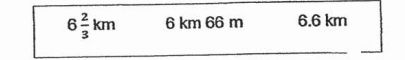# Primary 6 CA1 Practice #1

Time elapsed:
Q1. Which one of the following fractions is an equivalent fraction of 86 ?     [1 mark]
Q2. The shaded figure is formed by two identical circles with centres at A and C. ABCD is a square and length AB is 3.5 cm. Find the perimeter of the shaded figure. Take π = 227.     [2 marks]Q3. Which of the following has the same value as 45 + 3?     [1 mark]
Q4. A nylon rope is 67 m long. It is cut into 12 equal pieces. What is the length of each piece?     [1 mark]
Q5. The table below shows the charges for renting a bicycle on a weekend. Elias rented two bicycles from 1100 to 1500 on Sunday. How much did he pay altogether for renting the bicycles?     [1 mark]Q6. What does the digit 2 in 3,728,459 stand for?     [1 mark]
Q7. The capacity of 40 identical cups is 12.08 ℓ. What is the capacity of 1 such cup?     [2 marks]
Q8. Bryan is 56 as heavy as Xavier. What is the ratio of Xavier mass to their total mass?     [1 mark]
Q9. Find the value of 16.4 - 7.16.     [1 mark]
Q10. Simplify 24q - 8q + 3q.     [1 mark]
Q11. Shawn had 95 concert tickets. He gave 5 concert tickets to each of his friends and had 25 concert tickets left. How many friends did he give his concert tickets to?     [2 marks]
Q12. Arrange the following distance from the shortest to the longest.     [1 mark]Q13. Express 0.8 as a percentage.     [1 mark]
Q14. Sally and Gina baked 4 cakes each. 1 112 kg of flour was used for baking each cake. What was the total mass of flour used by both girls?     [2 marks]
Q15. Which one of the following fractions is not an equivalent fraction of 410?     [1 mark]
Q16. A bottle contained 29 litre of syrup. All the syrup was poured equally into 3 empty cups. How much syrup was there in each cup?     [1 mark]
Q17. There are 50 pupils in a class. 32 of them are boys. What percentage of the pupils are boys?     [1 mark]
Q18. In the number line below, what is the number indicated by the arrow?     [1 mark]
Q19. Find the value of 34 ÷ 67     [1 mark]
Q20. Which of the following is most likely to be the mass of a can of coke?     [1 mark]